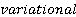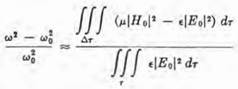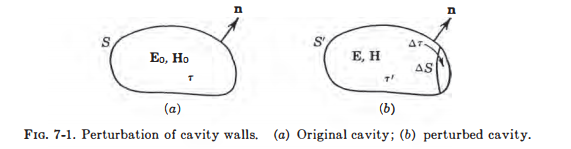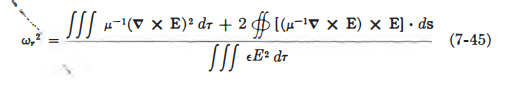# Consider a small deformation of the walls of a cavity, such as represented by Fig. 7-1. Take the  formula [Eq. (7-45)), which requires no boundary conditions on E, and take the unperturbed cavity field E0 as a trial field. Show that Eq. (7-45) reduces to Show that this formula is essentially the same as Eq. (7-4).

Question
1 views

Consider a small deformation of the walls of a cavity, such as represented by Fig. 7-1. Take theformula [Eq. (7-45)), which requires no boundary conditions on E, and take the unperturbed cavity field E0 as a trial field. Show that Eq. (7-45) reduces toShow that this formula is essentially the same as Eq. (7-4).### This question hasn't been answered yet.